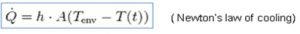# Cooling Power

### The FlexTC cooling power is a function of the Device Plunger selection and of the temperature transfer coefficient (here in after referred to as the “H factor”) between the touching surfaces as shown in the power dissipation formula:The higher the H factor is, the more powerful a thermal system can be, FlexTC systems H factor is very high, 4000(W·K¯¹·m¯² ) compared to the H factor of an air-stream-based systems which is only 120 (W·K¯¹·m¯²). As a consequence of which FlexTC systems can effectively cool high power device while air-stream-based systems cannot.

Qwatt = H x Asurface (Tcase – Tambient)
Q (watt) = The amount of dissipation, in watts, that a thermal system can dissipate.
H (W·K¯¹·m¯² )= Temperature transfer coefficient.
A (m²) = the surface area of the device
Tcase (°K) = the temperature of the device
Tambient (°K) = the temperature of the device plunger